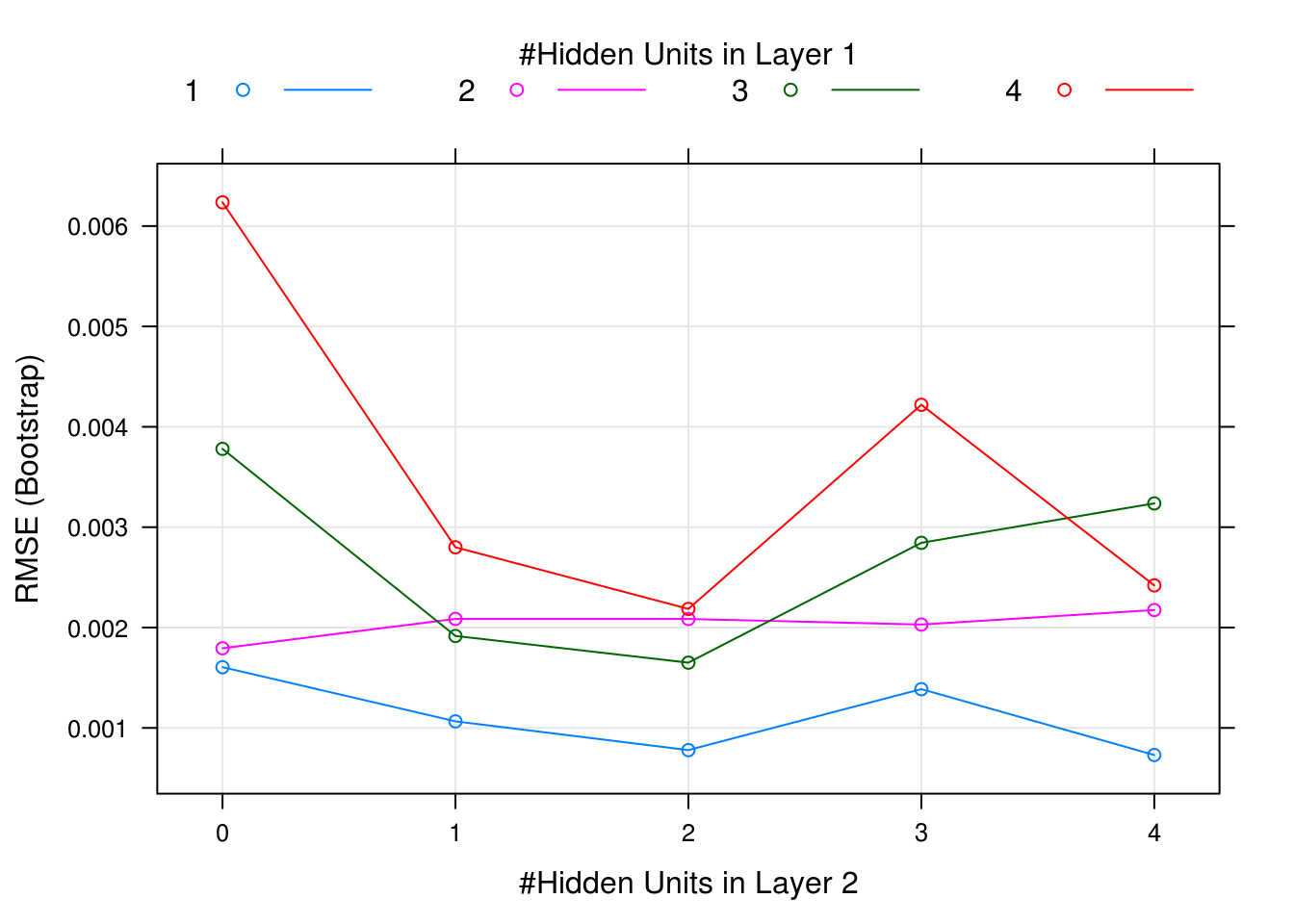## Neural Network Example

Read all the libraryies you need.

### Build labels for classification

In `iris` dataset, there are three types of flowers, which means we’re now dealing with a multi-class classification.

``````xor <- model.matrix(~ Species, Data)
xor[,1] <- ifelse(xor[,2] %in% 0 & xor[,3] %in% 0, 1, 0)
colnames(xor) <- 'Speciessetosa'
``````##   Speciessetosa Speciesversicolor Speciesvirginica
## 1             1                 0                0
## 2             1                 0                0
## 3             1                 0                0
## 4             1                 0                0
## 5             1                 0                0
## 6             1                 0                0``````
``Data <- cbind(xor, Data[,-5])``

### Split data for training

``````set.seed(30)
DataFinal <- Data[sample(nrow(Data)),]

Size <- floor(0.7*nrow(Data))

TrainSet <- DataFinal[1:Size, ]
TestSet <- DataFinal[c(Size+1):nrow(Data), ]``````

### Train NN model

We can us `neuralnet()` to train a NN model. Also, the `train()` function from `caret` can help us tune parameters. We can plot the result to see which set of parameters is fit our data the best.

``````FormulaNN <- Speciessetosa + Speciesversicolor + Speciesvirginica ~ Sepal.Length + Sepal.Width + Petal.Length + Petal.Width

Model <- train(form=FormulaNN,
data=TrainSet,
method="neuralnet",
### Parameters for layers
tuneGrid = expand.grid(.layer1=c(1:4), .layer2=c(0:4), .layer3=c(0)),
### Parameters for optmization
learningrate = 0.01,
threshold = 0.01,
stepmax = 50000
)``````
``````## Warning in nominalTrainWorkflow(x = x, y = y, wts = weights, info =
## trainInfo, : There were missing values in resampled performance measures.``````
``plot(Model)``### Bulid best model

Build model based on the result from the plot above and plot the model.

``````BestModel <- neuralnet(formula = FormulaNN,
data = TrainSet,
hidden = c(1,2),
learningrate = 0.01,
threshold = 0.01,
stepmax = 50000

)

plot(BestModel, rep = 'best')``````